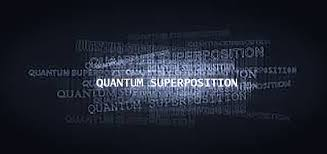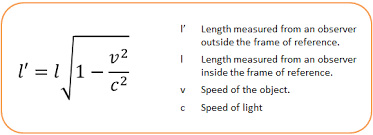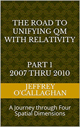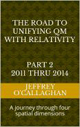# The classical “reality” of quantum Superposition

Superposition, in the quantum world means that on a quantum scale, particles can be thought of as waves that can exist in different states or positions at the same time.  Like all waves they can overlapping or be superimposed on each other and because it assumes that particles are waves, they can also exist in a superimposed state.  However, this means a particle can be in two places at once and in the quantum world only "decides" where it is and its "what" to be when it is observed.   This doesn’t make intuitive sense but it’s one of the weird realities of quantum physics.Many fell it is the only way to explain the experimental observations that support the superposition and wave particle duality is the non-classical non-intuitive one given by quantum mechanics.

However, Einstein unknowing may have able to define the classical "reality" of Superposition by extrapolating the rules of classical mechanics to the physical properties of space-time environment he defined.

One of the reasons he may have been unaware of this possibility is because superposition involves the spatial properties of position where as he chose define the universe in terms of time or the properties of four-dimensional space-time.  In other words, understanding the physical connection between the spatial properties of position and the time properties of Einstein space-time universe is extremely difficult for the same reasons as one would find it difficult to define a physical connection between apples and oranges.

However, Einstein gave us a way around this when he used the equation E=mc^2 and the constant velocity of light to define the geometric properties of mass and energy in a space-time universe because that provided a method of converting a unit of time he associated with energy in space-time to unit of space one can associate with four *spatial* dimensions.  Additionally, because the velocity of light is constant, he also defined a one to one quantitative correspondence between his space-time universe and one made up of four *spatial* dimensions.

This would allow one to understand the validity of quantum mechanics assumption that particles can be defined in terms of waves and how they can be superimposed or simultaneously be in multiple positions before being observed.

For example, the article, "Why is energy/mass quantized?" Oct. 4, 2007 showed that one can use the Einstein’s theories to explain and understand the physicality of the wave properties of particles by extrapolating the rules of classical resonance in a three-dimensional environment to a matter energy wave moving on “surface” of a three-dimensional space manifold with respect to a fourth *spatial* dimension.  It also explains why all energy must be quantized or exists in these discrete resonant systems when observed.

Briefly it showed the four conditions required for resonance to occur in a classical environment, an object, or substance with a natural frequency, a forcing function at the same frequency as the natural frequency, the lack of a damping frequency and the ability for the substance to oscillate spatial would occur in a matter wave moving in four *spatial* dimensions.

The existence of four *spatial* dimensions would give a matter energy wave the ability to oscillate spatially on a "surface" between a third and fourth *spatial* dimensions thereby fulfilling one of the requirements for classical resonance to occur.

These oscillations would be caused by an event such as the decay of a subatomic particle or the shifting of an electron in an atomic orbital.  This would force the "surface" of a three-dimensional space manifold with respect to a fourth *spatial* dimension to oscillate with the frequency associated with the energy of that event.

However, the oscillations caused by such an event would serve as forcing function allowing a resonant system or "structure" to be established in four spatial dimensions.

As was shown in that article these resonant systems in four *spatial* dimensions are responsible for the particle properties of matter.

However, one can also explain how the boundaries of a particle’s resonant structure are defined.

In classical physics, a point on the two-dimensional surface of paper is confined to that surface.  However, that surface can oscillate up or down with respect to three-dimensional space.

Similarly, an object occupying a volume of three-dimensional space would be confined to it however, it could, similar to the surface of the paper oscillate "up" or "down" with respect to a fourth *spatial* dimension.

It is the confinement of the "upward" and "downward" oscillations of a three-dimension volume with respect to a fourth *spatial* dimension which allows the resonate  structure the article "Why is energy/mass quantized?" Oct. 4, 2007 showed was responsible for a particle to exist.

In other words the when the matter energy wave is confined by an observation to three-dimensional space the interference between waves reflected back and forth by that confinement sets a resonant standing wave in space which is called a particle.

In other words, Einstein give us a classical validation of the quantum mechanical assumption that particles can be thought of as waves because it shows they are made up of resonate structure formed matter energy wave and why when someone observes its wave component it always appears as a particle.

Additionally, one of the most advantageous results of viewing the relativistic properties of Einstein’s theories in terms of their spatial instead of its time components is that gives us an answer to one of the most perplexing aspects of quantum mechanics; that of how and why a particle can simultaneously exists anywhere in the universe before being observed

This is because it tells the length of an object relative to another is effected by its relative velocity and that there are no preferred reference frames by which one can measure that length. Therefore, one must not only view the distance traversed by the wave with respect to an observer who was external to it but one must also view the distance between observers from the wave’s perspective. Yet it also tells us that the length of everything including the universe from an object or wave moving at the speed of light is zero as can be seen from his formal on the right for length contraction.Therefore, from the perspective of the energy wave the article "Why is energy/mass quantized?" showed was responsible for a particle which is moving at the speed of light with respect to all observers the distance or length between all observers no matter far they may be from their perspective is zero with respect to that wave.  Therefore, its energy exists at every point in between them.

This gives us an explanation in terms of physical properties of Einsteinâ€™s space-time universe for the VALIDITY of quantum mechanics assumption that a photon and its wave packet can simultaneously exists everywhere in in the universe before being observed. In other words, it only "decides" where it wants to be in space when it is prevented from moving at the speed of light relative to an observer by an observation.

However, viewing Einstein theories from the perceptive of their spatial instead of their time components also allows one to derive the classical reason why one must use probabilities to determine a particles position before being observed.

The fact that one can, as was show in the article mentioned earlier “Why is energy/mass quantized?” derive the particle properties of an energy wave as the result of a resonant structure formed on the "surface" of a three-dimensional space manifold with respect to a fourth *spatial* dimension also tells how a particle "decides" where it wants to be when observed in terms of our classical understanding of the world around us.

For example, the energy of a vibrating or oscillating ball on a rubber diaphragm would be disturbed over its entire surface while the magnitude of those vibrations would decrease as one move away from the focal point of the oscillations.

Similarly, if one accepts the validity of Einstein’s theories and the classical mechanism in the article “Why is energy/mass quantized?” which define a particle as result of resonant system created by vibrations or oscillations in a "surface" of three-dimensional space, those oscillations as was shown above would be distributed over the entire "surface" three-dimensional space with respect to all observers while the magnitude of those vibrations would be greatest at the focal point of the oscillations and decreases as one moves away from it.

Yet the science of Wave Mechanics tells us resonance would most probably occur on the surface of the rubber sheet were the magnitude of the vibrations is greatest and would diminish as one move away from that point,

Similarly, a particle would most probably be found were the magnitude of the vibrations in a "surface" of a three-dimensional space manifold is greatest and would diminish as one move away from that point.

This shows how one can make intuitive "sense" of Quantum Superposition and why the wave packet of a particle decides what and where it wants to be when observed  by extrapolating the rules of a classical mechanics to the spatial equivalent of Einstein’s theories.

It should be remembered Einstein genius allows us to view his theory in either four-dimensional space-time or its equivalent in only four *spatial* dimensions.  As was shown above changing one’s perspective on his theory from time to its spatial equivalent allows one to form an intuitive understanding of quantum Superposition based on our experiences in a three-dimensional world.

Latter Jeff

 The Road to Unifying QM with Relativity part 1 2007 thru 2010Ebook \$8.00Paper Back \$15.00 The Road to Unifying QM with Relativity part 2 2011 thru 2014Ebook \$8.00Paper Back \$16.00 The Road to Unifying QM with Relativity part 3 2015 thru 2020Ebook \$8.00Paper Back \$18.00